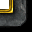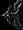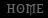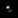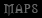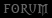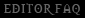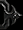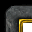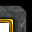# General - Generating Random Mazes

Tutorial By Pender

Automatic maze generation

by Pender

I. Introduction and Theory

There are many algorithms for generating mazes, and there are many kinds of mazes. If you want to read up on it generally, Wikipedia is a good place to start.

Our maze will use a modified version of Prim's Algorithm. Here are the steps of our general strategy, which I will then go through and explain how to implement:

1. Create a grid of impassable units.

I have chosen Scout Towers, which have an impassible area of 128x128.

Here is what they look like:However, this is how I will be representing them to you for clarity:In this picture, every gray square is a scout tower, and every green square is empty.

2. Add the scout tower at position (2,2) to a unit group, called in_cells, which I will mark in red.

Here is what I mean:3. Figure out which towers are exactly two spaces away from a red tower and put them in a unit group called frontier_cells, which I will mark in blue.5. Pick a random blue tower and mark it red.6. Pick a random red tower that is exactly two spaces away from the tower we just picked and add the tower between the two to a unit group called condemned_walls, which I will mark in black.7. Go back to step 3, and repeat until there are no more blue squares left.

Here is what a typical progression will look like:8. Remove all of the red and black towers.9. Remove the tower at (2,1) and the tower at (10,11).And that's the maze!

Here's what it looks like as actual towers:II. Implementation

Basically we will accomplish all of the above with five unit groups and clever usage of Pick Every Unit In Region.

As a side note, I think it is generally a better idea to load up the demo map and play with the triggers rather than read through the following mass of text. You should probably only use the rest of this section as a reference if you cannot understand what is going on in the demo map triggers.

1. Create a grid of impassable units.

This is easy. Start with an anchor point in the lower left, and use two nested For-loops to give you your grid.

The only tricky part is getting the lower-left anchor point, since it has to precisely line up with Warcraft III's internal grid or buildings will not sit exactly on them and the grid will get screwed up. I start off with a point that I place by hand, and then have the triggers place a single tower at that point. It will actually be slightly away from the point I placed by hand since buildings snap to the underlying grid. Then I set a point variable to the tower's actual location, remove the tower, and use that point as the anchor point. Here it is so far in code:

[SPOILER]Actions Set temp_group = (Units of type Scout Tower) Unit Group - Pick every unit in temp_group and do (Unit - Remove (Picked unit) from the game) Custom script: call DestroyGroup (udg_temp_group) Unit Group - Remove all units from Maze_Build_Frontier_cells Unit Group - Remove all units from Maze_Build_In_cells Unit Group - Remove all units from Maze_Build_Out_cells Unit Group - Remove all units from Maze_All_Units Unit Group - Remove all units from Maze_Build_Condemned_Walls Set Maze_Lower_Left = (Center of LowerLeft <gen>) Unit - Create 1 Scout Tower for Player 1 (Red) at Maze_Lower_Left facing Default building facing degrees Custom script: call RemoveLocation (udg_Maze_Lower_Left) Set Maze_Lower_Left = (Position of (Last created unit)) Unit - Remove (Last created unit) from the game For each (Integer A) from 0 to 10, do (Actions) Loop - Actions For each (Integer B) from 0 to 10, do (Actions) Loop - Actions Set temp_point = (Maze_Lower_Left offset by ((128.00 x (Real((Integer A)))), (128.00 x (Real((Integer B)))))) Unit - Create 1 Scout Tower for Player 1 (Red) at temp_point facing Default building facing degrees Custom script: call RemoveLocation (udg_temp_point) Unit Group - Add (Last created unit) to Maze_All_Units Unit Group - Add (Last created unit) to Maze_Build_Out_cells Set Current_Frontier_Cell_Coords = (Maze_Lower_Left offset by (128.00, 128.00)) Trigger - Run Begin mazing <gen> (checking conditions) [/SPOILER]

2. Add the scout tower at position (2,2) to a unit group, called in_cells, which I will mark in red.

Start at the lower left anchor position offset by two tower widths over and two tower widths up. This means the anchor point offset by (256, 256). Look for the tower there, and mark it red -- which means add it to the group Maze_Build_In_Cells.

[SPOILER]Actions Set temp_region = (Region centered at Current_Frontier_Cell_Coords with size (64.00, 64.00)) Set temp_group = (Units in temp_region matching (((Matching unit) is in Maze_All_Units) Equal to True)) Unit Group - Pick every unit in temp_group and do (Actions) Loop - Actions Unit Group - Add (Picked unit) to Maze_Build_In_cells Unit Group - Remove (Picked unit) from Maze_Build_Out_cells Custom script: call DestroyGroup (udg_temp_group) Custom script: call RemoveRect(udg_temp_region) [/SPOILER]

You may be wondering why I use variable regions instead of ranges. The reason is that they are computationally easier for Warcraft to handle, even though they are more of a pain to clean up afterwards to prevent leaks. Computing ranges involves a square root function, whereas regions, being rectangular, involve only less-than / greater-than comparisons. This doesn't make a difference on a 10x10 maze, but it may on larger ones.

3. Figure out which towers are exactly two spaces away from a red tower and put them in a unit group called frontier_cells, which I will mark in blue.

This is accomplished with another for-loop, from 0 to 3, which uses the polar-offset function to look in each of four directions for towers to mark blue -- which means add to the group Maze_Build_Frontier_Cells.

[SPOILER]Actions ... For each (Integer A) from 0 to 3, do (Actions) Loop - Actions Set temp_point = (Current_Frontier_Cell_Coords offset by 256.00 towards ((Real((Integer A))) x 90.00) degrees) Set temp_region = (Region centered at temp_point with size (64.00, 64.00)) Set temp_group = (Units in temp_region matching (((Matching unit) is in Maze_All_Units) Equal to True)) Unit Group - Pick every unit in temp_group and do (Unit Group - Add (Picked unit) to Maze_Build_Frontier_cells) Custom script: call RemoveLocation (udg_temp_point) Custom script: call RemoveRect(udg_temp_region) Custom script: call DestroyGroup (udg_temp_group) Trigger - Run Pick frontier cell and iterate <gen> (checking conditions) [/SPOILER]

5. Pick a random blue tower and mark it red.

OK, now here we transition to our heavy-lifter trigger, "Pick Frontier Cell and Iterate."

6. Pick a random red tower that is exactly two spaces away from the tower we just picked and add the tower between the two to a unit group called condemned_walls, which I will mark in black.

For this part, we need to create another unit group -- frontier_cell_in_neighbors -- in which to count up all the in (red) cells near our chosen cell so that we can then pick one of them randomly and condemn the wall between them.

To find the wall, just average the x and y components of the locations of the chosen frontier cell and the chosen in cell and there you will find the wall.

[SPOILER]Actions Set Current_Frontier_Cell_Coords = (Position of (Random unit from Maze_Build_Frontier_cells)) Set temp_region = (Region centered at Current_Frontier_Cell_Coords with size (64.00, 64.00)) Set temp_group = (Units in temp_region matching (((Matching unit) is in Maze_Build_Frontier_cells) Equal to True)) Unit Group - Pick every unit in temp_group and do (Actions) Loop - Actions Unit Group - Add (Picked unit) to Maze_Build_In_cells Unit Group - Remove (Picked unit) from Maze_Build_Out_cells Unit Group - Remove (Picked unit) from Maze_Build_Frontier_cells Custom script: call DestroyGroup (udg_temp_group) Custom script: call RemoveRect(udg_temp_region) -------- Note: frontier_cell_in_neighbors does not leak because it is never created with a "pick units" action; units are added and subtracted piecemeal. Destroygrouping it breaks the trigger. -------- Unit Group - Remove all units from frontier_cell_in_neighbors For each (Integer A) from 0 to 3, do (Actions) Loop - Actions Set temp_point = (Current_Frontier_Cell_Coords offset by 256.00 towards ((Real((Integer A))) x 90.00) degrees) Set temp_region = (Region centered at temp_point with size (128.00, 128.00)) Set temp_group = (Units in temp_region matching (((Matching unit) is in Maze_Build_In_cells) Equal to True)) Unit Group - Pick every unit in temp_group and do (Unit Group - Add (Picked unit) to frontier_cell_in_neighbors) Custom script: call RemoveLocation (udg_temp_point) Custom script: call RemoveRect(udg_temp_region) Custom script: call DestroyGroup (udg_temp_group) Set Current_In_Cell_Coords = (Position of (Random unit from frontier_cell_in_neighbors)) Set temp_point = (Point((((X of Current_Frontier_Cell_Coords) + (X of Current_In_Cell_Coords)) / 2.00), (((Y of Current_Frontier_Cell_Coords) + (Y of Current_In_Cell_Coords)) / 2.00))) Set temp_region = (Region centered at temp_point with size (128.00, 128.00)) Set temp_group = (Units in temp_region matching (((Matching unit) is in Maze_All_Units) Equal to True)) Unit Group - Pick every unit in temp_group and do (Unit Group - Add (Picked unit) to Maze_Build_Condemned_Walls) Custom script: call RemoveLocation (udg_temp_point) Custom script: call RemoveRect(udg_temp_region) Custom script: call DestroyGroup (udg_temp_group) For each (Integer A) from 0 to 3, do (Actions) Loop - Actions Set temp_point = (Current_Frontier_Cell_Coords offset by 256.00 towards ((Real((Integer A))) x 90.00) degrees) Set temp_region = (Region centered at temp_point with size (128.00, 128.00)) Set temp_group = (Units in temp_region matching (((Matching unit) is in Maze_Build_Out_cells) Equal to True)) Unit Group - Pick every unit in temp_group and do (Unit Group - Add (Picked unit) to Maze_Build_Frontier_cells) Custom script: call RemoveLocation (udg_temp_point) Custom script: call RemoveRect(udg_temp_region) Custom script: call DestroyGroup (udg_temp_group) Custom script: call RemoveLocation (udg_Current_In_Cell_Coords) Custom script: call RemoveLocation (udg_Current_Frontier_Cell_Coords) [/SPOILER]

7. Go back to step 3, and repeat until there are no more blue squares left.

This is accomplished by having our trigger, "Pick Frontier Cell and Iterate" trigger itself if there are more frontier cells, and trigger "Finish Up" if not.

... If ((Maze_Build_Frontier_cells is empty) Equal to True) then do (Trigger - Run Finish Up <gen> (checking conditions)) else do (Trigger - Run (This trigger) (checking conditions))

8. Remove all of the red and black towers.

9. Remove the tower at (2,1) and the tower at (10,11).

These two are implemented by our "Finish Up" trigger:

[SPOILER]Actions Unit Group - Pick every unit in Maze_Build_In_cells and do (Unit - Remove (Picked unit) from the game) Unit Group - Pick every unit in Maze_Build_Condemned_Walls and do (Unit - Remove (Picked unit) from the game) Set temp_point = (Maze_Lower_Left offset by (128.00, 0.00)) Set temp_region = (Region centered at temp_point with size (64.00, 64.00)) Set temp_group = (Units in temp_region matching (((Matching unit) is in Maze_All_Units) Equal to True)) Unit Group - Pick every unit in temp_group and do (Unit - Remove (Picked unit) from the game) Custom script: call RemoveLocation (udg_temp_point) Custom script: call RemoveRect(udg_temp_region) Custom script: call DestroyGroup (udg_temp_group) Set temp_point = (Maze_Lower_Left offset by ((128.00 x 9.00), (128.00 x 10.00))) Set temp_region = (Region centered at temp_point with size (64.00, 64.00)) Set temp_group = (Units in temp_region matching (((Matching unit) is in Maze_All_Units) Equal to True)) Unit Group - Pick every unit in temp_group and do (Unit - Remove (Picked unit) from the game) Custom script: call RemoveLocation (udg_temp_point) Custom script: call RemoveRect(udg_temp_region) Custom script: call DestroyGroup (udg_temp_group) [/SPOILER]

Here is the end result:If you want to see the same thing, load up the (unprotected) demo map attached and type "-go" .

III. Elaboration

From here, the possibilities are endless. For an example of a polished multiplayer map built around random mazes, check out The Labyrinth, attached. Here is a typical screenshot from that map:You can also make the maze substantially larger, up to 27x27 before you start running into unit limits. To create a 27x27 maze in the demo map, type "-big". It looks like this:There is also no reason the towers have to start out as a giant square. Any overall shape will do, so long as they are right up next to one another. I hacked together a quick demonstration of a circular maze in the attached demo map; to access it, type "-circle" . It has no border, but it is a demonstration of the concept.Consider replacing the towers with destructibles like trees or rocks, or have a trigger paint the ground that they're on as a different tile before deleting them. Then you can test if a unit has wandered off of the grass terrain type and wreak vengeance upon them if so. The demo map has commands for both of these; to access them, type either "-terrain" or "-hedge" after you have created a maze with one of the other commands. They look like this:IV. Using Variables Instead of Towers

What we have so far is, frankly, a hack. Creating a ton of towers and then figuring out which ones are proximate to other ones with regions is enormously computationally expensive and runs into unit limits fairly quickly.

Ideally, we would take this approach and abstract it all into variables. No towers, no unit groups, no regions: all that would remain is a few lists of integers that Warcraft III manipulates into a maze and then drops onto the map all at once.

Here is a list of roadblocks we'll face in translating our physical approach into a pure variable approach, and how to overcome them:

1. Warcraft III does not support multi-dimensional arrays.

You can have a one-dimensional array of the form Array[x], but, ridiculously, you can't have a two-dimensional array of the form Array[x][y]. My handheld graphing calculator can handle multi-dimensional arrays, but not Warcraft III.

We get around this by using an extra-long one-dimensional array and "imputing" an extra dimension. To create a 51x51 virtual array, create an integer array of length 51^2, or 2601. Then, to access the virtual coordinates V_Array[x][y], instead call Array[51*x + y].

2. When we used units, we had Unit Groups. What do we use here?

This is the hardest part of the translation from units to variables. First, we'll use the integer values in our master grid to denote the state of that cell. I used the following key:

• 0 = out-cell
• 1 = in-cell
• 2 = frontier cell
• 3 = condemned wall

Previously, we could tell warcraft to pick a random frontier cell. With integers instead of units, we'll make a list of the index numbers of the frontier cells and then pick one randomly with a random integer.

The brute-force way to accomplish this goal is to run through all 2601 items of your master grid every iteration and make a list of all the items currently set to "2". The problem is that it is dog slow -- slow enough to consume a lot of the time saved by using variables instead of units.

The much better solution is to keep a single dynamic list. Every time you add a frontier cell to the list, you attach its index number to the end and bump the variable that keeps track of the array length. Every time you remove a frontier cell from the list, you subtract one from the variable that keeps track of the array length and then cycle from that item through to the end of the array, setting each item to the value of the following item. That way, you can remove a single item from the middle of the array, and all the following items move down to fill the hole.

3. You have to keep track of the maze edges yourself.

With units, Warcraft would treat the edges elegantly, since it would proceed calmly forward if it found no tower unit where you asked it to look. Here, things would start to fall apart if we tested units outside the bounds of our array. Especially because it is a virtual 2-dimensional array, we'd start referencing unrelated items if our coordinates were out of bounds. So when we run a check for neighboring out-cells to mark as frontier cells, we have to make sure the neighbor we're talking about is not out of bounds. The problem is easily enough handled, but you have to know to watch out for it.

Okay! Those are all the problems and their solutions.

Here is our finished code:

[SPOILER]Create grid of virtual units:Actions Set Virtual_iteration_counter = 0 Set Virtual_frontier_list_counter = 0 -------- Cleanup & preliminaries -------- Unit - Move Footman 0001 <gen> instantly to start_loc, facing 90.00 degrees Set temp_group = (Units of type Scout Tower) Unit Group - Pick every unit in temp_group and do (Unit - Remove (Picked unit) from the game) Custom script: call DestroyGroup (udg_temp_group) Destructible - Pick every destructible in (Playable map area) and do (Destructible - Remove (Picked destructible)) Set temp_point = (Center of (Playable map area)) Environment - Change terrain type at temp_point to Lordaeron Summer - Grass using variation -1 in an area of size 1000 and shape Square Custom script: call RemoveLocation (udg_temp_point) Set Maze_Lower_Left = (Center of LowerLeft <gen>) Unit - Create 1 Scout Tower for Player 1 (Red) at Maze_Lower_Left facing Default building facing degrees Custom script: call RemoveLocation (udg_Maze_Lower_Left) Set Maze_Lower_Left = (Position of (Last created unit)) Unit - Remove (Last created unit) from the game -------- Create virtual grid -------- -------- This grid is 51x51. To access (x,y) (starting at (0,0)), get item 51x + y. -------- -------- Key: 0 = out; 1 = in; 2 = frontier; 3 = condemned wall -------- For each (Integer A) from 0 to 2600, do (Actions) Loop - Actions Set Virtual_Grid[(Integer A)] = 0 -------- Mark (25,25) as "in" -------- Set Virtual_current_frontier_X = 25 Set Virtual_current_frontier_Y = 25 Set Virtual_Grid[((51 x Virtual_current_frontier_X) + Virtual_current_frontier_Y)] = 1 -------- Mark in-cells two units away as "frontier" -------- If (All Conditions are True) then do (Then Actions) else do (Else Actions) If - Conditions (Virtual_current_frontier_X + 2) Less than or equal to 50 Then - Actions Set Virtual_Grid[((51 x (Virtual_current_frontier_X + 2)) + Virtual_current_frontier_Y)] = 2 Set Virtual_list_of_frontier_cells[Virtual_frontier_list_counter] = ((51 x (Virtual_current_frontier_X + 2)) + Virtual_current_frontier_Y) Set Virtual_frontier_list_counter = (Virtual_frontier_list_counter + 1) Else - Actions If (All Conditions are True) then do (Then Actions) else do (Else Actions) If - Conditions (Virtual_current_frontier_X - 2) Greater than or equal to 0 Then - Actions Set Virtual_Grid[((51 x (Virtual_current_frontier_X - 2)) + Virtual_current_frontier_Y)] = 2 Set Virtual_list_of_frontier_cells[Virtual_frontier_list_counter] = ((51 x (Virtual_current_frontier_X - 2)) + Virtual_current_frontier_Y) Set Virtual_frontier_list_counter = (Virtual_frontier_list_counter + 1) Else - Actions If (All Conditions are True) then do (Then Actions) else do (Else Actions) If - Conditions (Virtual_current_frontier_Y + 2) Less than or equal to 50 Then - Actions Set Virtual_Grid[((51 x Virtual_current_frontier_X) + (Virtual_current_frontier_Y + 2))] = 2 Set Virtual_list_of_frontier_cells[Virtual_frontier_list_counter] = ((51 x Virtual_current_frontier_X) + (Virtual_current_frontier_Y + 2)) Set Virtual_frontier_list_counter = (Virtual_frontier_list_counter + 1) Else - Actions If (All Conditions are True) then do (Then Actions) else do (Else Actions) If - Conditions (Virtual_current_frontier_Y - 2) Greater than or equal to 0 Then - Actions Set Virtual_Grid[((51 x Virtual_current_frontier_X) + (Virtual_current_frontier_Y - 2))] = 2 Set Virtual_list_of_frontier_cells[Virtual_frontier_list_counter] = ((51 x Virtual_current_frontier_X) + (Virtual_current_frontier_Y - 2)) Set Virtual_frontier_list_counter = (Virtual_frontier_list_counter + 1) Else - Actions -------- And off we go! -------- Trigger - Run Pick virtual frontier cell and iterate <gen> (checking conditions)

Pick virtual frontier cell and iterate:Actions Set Virtual_iteration_counter = (Virtual_iteration_counter + 1) If ((Virtual_iteration_counter mod 300) Equal to 0) then do (Wait 0.01 seconds) else do (Do nothing) -------- Have we run out of frontier cells? -------- If (All Conditions are True) then do (Then Actions) else do (Else Actions) If - Conditions Virtual_frontier_list_counter Greater than 0 Then - Actions -------- Not yet. -------- -------- Choose a random cell from the list as the current frontier cell -------- Set temp_random_int = (Random integer number between 0 and (Virtual_frontier_list_counter - 1)) Set temp_integer = Virtual_list_of_frontier_cells[temp_random_int] Set Virtual_current_frontier_X = ((temp_integer - (temp_integer mod 51)) / 51) Set Virtual_current_frontier_Y = (temp_integer mod 51) -------- Mark the current frontier cell as "in" -------- Set Virtual_Grid[temp_integer] = 1 -------- Remove the current frontier cell from the list of frontier cells -------- Set Virtual_frontier_list_counter = (Virtual_frontier_list_counter - 1) For each (Integer A) from temp_random_int to (Virtual_frontier_list_counter - 1), do (Actions) Loop - Actions Set Virtual_list_of_frontier_cells[(Integer A)] = Virtual_list_of_frontier_cells[((Integer A) + 1)] -------- Cycle through the neighboring cells, listing the ins and marking the outs as frontiers -------- Set Virtual_In_Neighbor_Counter = 0 If (All Conditions are True) then do (Then Actions) else do (Else Actions) If - Conditions (Virtual_current_frontier_X + 2) Less than or equal to 50 Then - Actions Set temp_integer = ((51 x (Virtual_current_frontier_X + 2)) + Virtual_current_frontier_Y) Trigger - Run List in or turn out into frontier <gen> (checking conditions) Else - Actions If (All Conditions are True) then do (Then Actions) else do (Else Actions) If - Conditions (Virtual_current_frontier_X - 2) Greater than or equal to 0 Then - Actions Set temp_integer = ((51 x (Virtual_current_frontier_X - 2)) + Virtual_current_frontier_Y) Trigger - Run List in or turn out into frontier <gen> (checking conditions) Else - Actions If (All Conditions are True) then do (Then Actions) else do (Else Actions) If - Conditions (Virtual_current_frontier_Y + 2) Less than or equal to 50 Then - Actions Set temp_integer = ((51 x Virtual_current_frontier_X) + (Virtual_current_frontier_Y + 2)) Trigger - Run List in or turn out into frontier <gen> (checking conditions) Else - Actions If (All Conditions are True) then do (Then Actions) else do (Else Actions) If - Conditions (Virtual_current_frontier_Y - 2) Greater than or equal to 0 Then - Actions Set temp_integer = ((51 x Virtual_current_frontier_X) + (Virtual_current_frontier_Y - 2)) Trigger - Run List in or turn out into frontier <gen> (checking conditions) Else - Actions -------- Choose a random cell from the new list as the current in-cell -------- Set temp_integer = Virtual_In_Neighbor_List[(Random integer number between 0 and (Virtual_In_Neighbor_Counter - 1))] Set Virtual_current_in_X = ((temp_integer - (temp_integer mod 51)) / 51) Set Virtual_current_in_Y = (temp_integer mod 51) Set temp_integer = ((51 x ((Virtual_current_in_X + Virtual_current_frontier_X) / 2)) + ((Virtual_current_in_Y + Virtual_current_frontier_Y) / 2)) Set Virtual_Grid[temp_integer] = 3 -------- Now iterate. -------- Trigger - Run (This trigger) (checking conditions) Else - Actions -------- Yes. Finish up. -------- Trigger - Run Finish up fast maze <gen> (checking conditions)

IMPORTANT: Note the "wait" command near the top of this action. That is necessary to prevent the map from crashing on my computer. My conjecture is that if you freeze up the game with pure computation for too long -- without modifying units or otherwise making the game think it's playing -- either the game or the operating system thinks it's frozen and it triggers a crash recovery. If you experience crashiness, try tinkering with that value: lower the "mod" number from 300 to, say, 100 or 50.

List in or turn out into frontier:Actions -------- Is it an in-cell? -------- If (All Conditions are True) then do (Then Actions) else do (Else Actions) If - Conditions Virtual_Grid[temp_integer] Equal to 1 Then - Actions -------- Add it to the list. -------- Set Virtual_In_Neighbor_List[Virtual_In_Neighbor_Counter] = temp_integer Set Virtual_In_Neighbor_Counter = (Virtual_In_Neighbor_Counter + 1) Else - Actions -------- Is it an out-cell? -------- If (All Conditions are True) then do (Then Actions) else do (Else Actions) If - Conditions Virtual_Grid[temp_integer] Equal to 0 Then - Actions -------- Mark it "frontier." -------- Set Virtual_Grid[temp_integer] = 2 Set Virtual_list_of_frontier_cells[Virtual_frontier_list_counter] = temp_integer Set Virtual_frontier_list_counter = (Virtual_frontier_list_counter + 1) Else - Actions

Finish up fast maze:Actions Set Virtual_Grid[((51 x 1) + 0)] = 3 Set Virtual_Grid[((51 x 49) + 50)] = 3 For each (Integer A) from 0 to 2600, do (Actions) Loop - Actions If (All Conditions are True) then do (Then Actions) else do (Else Actions) If - Conditions Virtual_Grid[(Integer A)] Equal to 0 Then - Actions Set temp_point = (Maze_Lower_Left offset by ((Real((128 x (((Integer A) - ((Integer A) mod 51)) / 51)))), (Real((128 x ((Integer A) mod 51)))))) Environment - Change terrain type at temp_point to Lordaeron Summer - Dirt using variation -1 in an area of size 1 and shape Square Custom script: call RemoveLocation (udg_temp_point) Else - Actions [/SPOILER]

Now we have a super-fast maze-generation system that is not bound by unit limits. If you'd like to see it in action, load up the attached demo map (updated as of 4/22/08) and type "-fastmaze". In just a second or two, the map will create a 51x51 maze -- around four times the size of what is practically possible with the unit method, and in a fraction of the time. Here is what it looks like:I hope you enjoyed the tutorial. I also hope this will encourage you not to use a hand-placed maze in your map if you can make the same thing with the randomized kind this tutorial is all about.